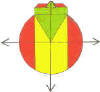DMD   EDU-HOME

FORMULAE AND ALGORITHMS

PROBLEM  1:

Zanele can paint a room in 2 hours, while Thabo can paint the same room in 3 hours.  How long will they take to paint this room working together, and assuming that they work at the same rate?

SOLUTION

Zanele’s work rate is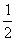of the room per hour, while Thabo’s work rate is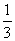of the room per hour.  Their combined work rate isth of the room per hour.

NOW DO THE INVERSE:

What is their joint time taken to paint the whole room? That is, what is the time taken per room?

If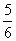th of the room is painted in one hour, then

1 (the whole) room will be painted in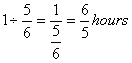= 1.2 hours (or 1hour 12 minutes)

THE FORMULA:

TIME TO PAINT THE ROOM  =NOTE:         There is a step by step process or procedure, called an ALGORITHM whereby one could obtain the formula to solve the above problem. This process involves reading, deep understanding and proper analysis of the problem. 0f course, knowing which formula to use also requires insight and understanding of the problem in itself.

The algorithm:

•                       Determine the work rate. (Portion of room painted per hour.)

•                       Do the inverse: time taken per room.

PROBLEM  2 :  PERCENTAGES

If x percent of a certain total represents y, what is the total.

FORMULA:              TOTAL  =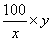ALGORITHM:           If                   x % of the total represents y

therefore,      100 % represents …..?

OR: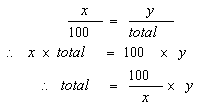©  DESMOND DESAI, DMD EDU-HOME, 2007

All rights reserved. Designed and created by Desmond Desai, South Africa. This page is protected by Copyright. No part of this page may be reproduced, stored in a retrieval system, or transmitted in any form of by any means, electronic, mechanical, photocopying, recording or otherwise, without the prior written permission of the copyright holder.